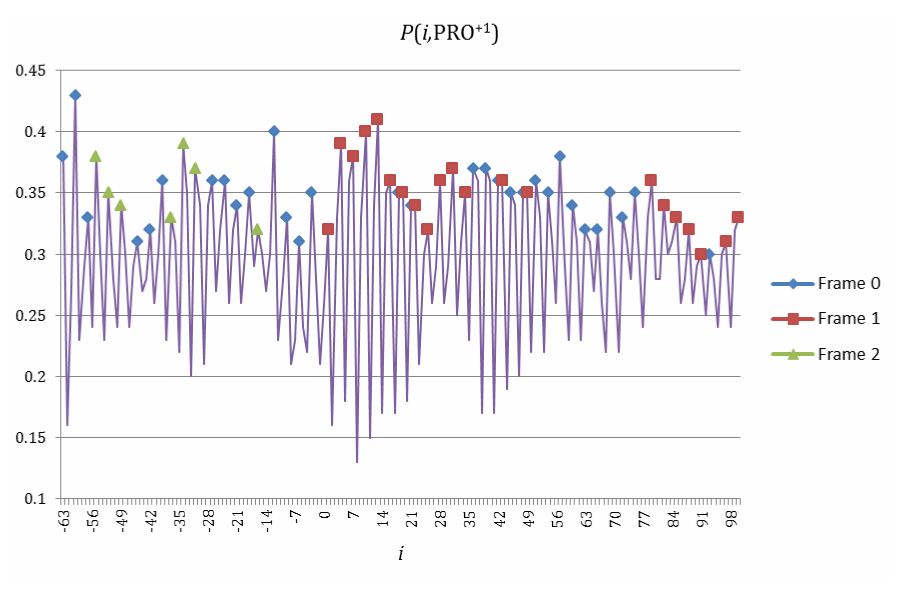Figure 7: The score function P(i, PRO+1) (Formula 2) computed on +1 frameshift of prokaryotic genes PRO+1. Before the frameshift site, the majority of local peaks (y = 14, n = 21, y/n = 67%, a = 0.040% with Formula 4) are in frame 0 (rhombuses). After the frameshift site, the majority of local peaks (y = 21, n = 34, y/n = 62%, a = 0.018% with Formula 4) are in frame 1 (squares).